X-ray Photoelectron Spectroscopy

BACKGROUND

X-ray Photoelectron spectroscopy utilizes photo-ionization and energy-dispersive analysis of the emitted photoelectrons to study the composition and electronic state of the surface region of a sample.

X-ray Photoelectron spectroscopy is based upon a single photon in/electron out process and from many viewpoints this underlying process is a much simpler phenomenon than the Auger process.

The energy of a photon is given by the Einstein relation :

E = hn

where

h - Plank's constant ( 6.62x10-34 J s )
n - frequency (Hz) of the radiation

In XPS the photon is absorbed by an atom in a molecule or solid, leading to ionization and the emission of a core (inner shell) electron. By contrast, in UPS the photon interacts with valence levels of the molecule or solid, leading to ionisation by removal of one of these valence electrons.

The kinetic energy distribution of the emitted photoelectrons can be measured using any appropriate electron energy analyser and a photoelectron spectrum can thus be recorded.

The process of photoionization can be considered in several ways : one way is to look at the overall process as follows :

A + hn --> A+ + e-

Conservation of energy then requires that :

E(A) + hn = E(A+) + E(e-)

Since the electron's energy is present solely as kinetic energy (KE) this can be rearranged to give the following expression for the KE of the photoelectron :

KE = hn - (E(A+) - E(A))

The final term in brackets, representing the difference in energy between the ionized and neutral atoms, is generally called the binding energy (BE) of the electron - this then leads to the following commonly quoted equation :

KE = hn - BE

An alternative approach is to consider a one-electron model along the lines of the following pictorial representation ; this model of the process has the benefit of simplicity but it can be rather misleading.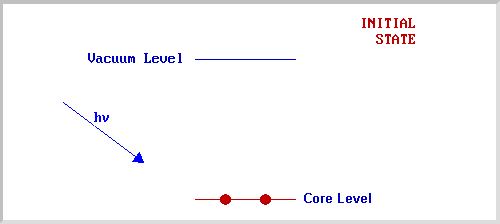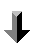The BE is now taken to be a direct measure of the energy required to just remove the electron concerned from its initial level to the vacuum level and the KE of the photoelectron is again given by :

KE = hn - BE

NOTE - The binding energies (BE) of energy levels in solids are conventionally measured with respect to the Fermi-level of the solid, rather than the vacuum level. This involves a small correction to the equation given above in order to account for the work function (F) of the solid, but for the purposes of the discussion below this correction will be neglected.

For each and every element, there will be a characteristic binding energy associated with each core atomic orbital, i.e. each element will give rise to a characteristic set of peaks in the photoelectron spectrum at kinetic energies determined by the photon energy and the respective binding energies.

The presence of peaks at particular energies therefore indicates the presence of a specific element in the sample under study, furthermore, the intensity of the peaks is related to the concentration of the element within the sampled region. Thus, the technique is capable of yielding a quantitative analysis and is sometimes known by the alternative acronym, ESCA (Electron Spectroscopy for Chemical Analysis).

The most commonly employed X-ray sources are those giving rise to:

Mg Ka radiation : hn = 1253.6 eV

Al Ka radiation : hn = 1486.6 eV

The emitted photoelectrons will therefore have kinetic energies in the range of ca. 0 - 1250 eV or 0 - 1480 eV . Since such electrons have very short IMFPs in solids, the technique is necessarily surface sensitive.

Example 1 - the XPS spectrum of NaCl

The diagram opposite shows an energy level diagram for sodium with approximate binding energies for the core levels.Example 2 - the XPS spectrum of Pd metal

The diagram below shows a real XPS spectrum obtained from a Pd metal sample using Mg Ka radiation.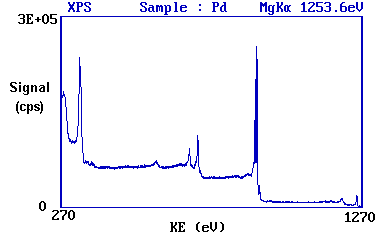The main peaks occur at kinetic energies of ca. 330, 690, 720, 910 and 920 eV.

Since the energy of the radiation is known, it is a trivial matter to transform the spectrum so that it is plotted against BE as opposed to KE.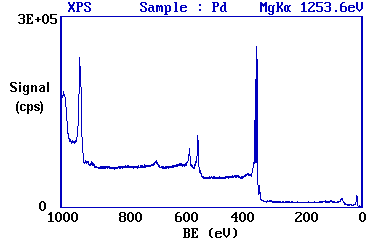The most intense peak is now seen to occur at a binding energy of ca. 335 eV

Working downwards from the highest energy levels ......

1. The valence band (4d,5s) emission occurs at a binding energy of ca. 0 - 8 eV (measured with respect to the Fermi level, or alternatively at ca. 4 - 12 eV if measured with respect to the vacuum level).

2. The emission from the 4p and 4s levels gives rise to very weak peaks at 54 and 88 eV, respectively.

3. The most intense peak at ca. 335 eV is due to emission from the 3d levels of the Pd atoms, while the 3p and 3s levels give rise to the peaks at ca. 534/561 eV and 673 eV, respectively.

4. The remaining peak is not an XPS peak at all, but it is an Auger peak arising from X-ray induced Auger emission. It occurs at a kinetic energy of ca. 330 eV (in this case it is really meaningless to refer to an associated binding energy).

These assignments are summarised below …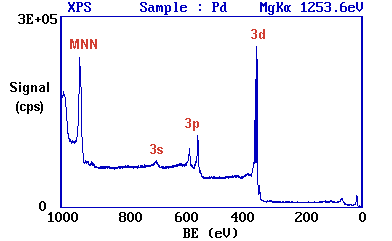It may be further noted that There are significant differences in the natural widths of the various photoemission peaks the peak intensities are not simply related to the electron occupancy of the orbitals.

Spin-Orbit Splitting

Closer inspection of the spectrum shows that emission from some levels (most obviously 3p and 3d) does not give rise to a single photoemission peak, but a closely spaced doublet.

We can see this more clearly if, for example, we expand the spectrum in the region of the 3d emission ...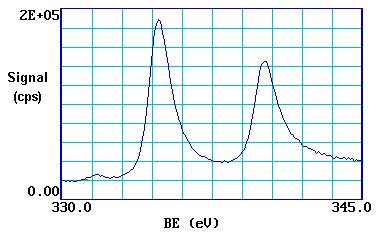The 3d photoemission is in fact split between two peaks, one at 334.9 eV BE and the other at 340.2 eV BE, with an intensity ratio of 3:2. This arises from spin-orbit coupling effects in the final state. The inner core electronic configuration of the initial state of the Pd is:

(1s)2(2s)2(2p)6(3s)2(3p)6(3d)10 ....

with all sub-shells completely full.

The removal of an electron from the 3d sub-shell by photo-ionization leads to a (3d)9 configuration for the final state - since the d-orbitals (l = 2) have non-zero orbital angular momentum, there will be coupling between the unpaired spin and orbital angular momenta.

Spin-orbit coupling is generally treated using one of two models which correspond to the two limiting ways in which the coupling can occur - these being the LS (or Russell-Saunders) coupling approximation and the j-j coupling approximation.

If we consider the final ionized state of Pd within the Russell-Saunders coupling approximation, the (3d)9 configuration gives rise to two states (ignoring any coupling with valence levels) which differ slightly in energy and in their degeneracy ...

2D 5/2 g J = 2x{5/2}+1 = 6

2D 3/2 g J = 2x{3/2}+1 = 4

These two states arise from the coupling of the L=2 and S=1/2 vectors to give permitted J values of 3/2 and 5/2. The lowest energy final state is the one with maximum J (since the shell is more than half full), i.e. J = 5/2, hence this gives rise to the "lower binding energy" peak. The relative intensities of the two peaks reflects the degeneracies of the final states (g J = 2J+1), which in turn determines the probability of transition to such a state during photoionization.

The Russell-Saunders coupling approximation is best applied only to light atoms and this splitting can alternatively be described using individual electron l-s coupling. In this case the resultant angular momenta arise from the single hole in the d-shell; a d-shell electron (or hole) has l = 2 and s = ˝, which again gives permitted j-values of 3/2 and 5/2 with the latter being lower in energy.

The peaks themselves are conventionally annotated as indicated - note the use of lower case lettering.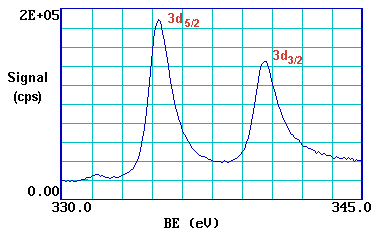This spin-orbit splitting is of course not evident with s-levels (l = 0), but is seen with p,d & f core-levels which all show characteristic spin-orbit doublets.

Chemical Shifts

The exact binding energy of an electron depends not only upon the level from which photoemission is occurring, but also upon:

1. The formal oxidation state of the atom.
2. The local chemical and physical environment.

Changes in either (1) or (2) give rise to small shifts in the peak positions in the spectrum, the so-called chemical shifts. Such shifts are readily observable and interpretable in XPS spectra (unlike in Auger spectra) because the technique: is of high intrinsic resolution (as core levels are discrete and generally of a well-defined energy) is a one electron process (thus simplifying the interpretation)

Atoms of a higher positive oxidation state exhibit a higher binding energy due to the extra coulombic interaction between the photo-emitted electron and the ion core. This ability to discriminate between different oxidation states and chemical environments is one of the major strengths of the XPS technique.

In practice, the ability to resolve between atoms exhibiting slightly different chemical shifts is limited by the peak widths which are governed by a combination of factors, especially the intrinsic width of the initial level and the lifetime of the final state the line-width of the incident radiation - which for traditional x-ray sources can only be improved by using X-ray monochromators the resolving power of the electron-energy analyser

In most cases, the second factor is the major contribution to the overall line width.

Examples of chemical shifts

Example 1: Oxidation States of Titanium

Titanium exhibits very large chemical shifts between different oxidation states of the metal; in the diagram below a Ti 2p spectrum from the pure metal (Ti) is compared with a spectrum of titanium dioxide (Ti4+).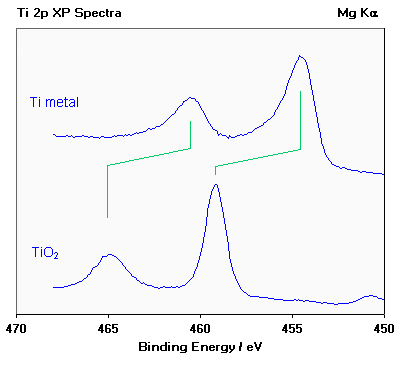Note :

(i) the two spin orbit components exhibit the same chemical shift (~ 4.6 eV);
(ii) metals are often characterized by an asymmetric line shape, with the peak tailing to higher binding energy, while insulating oxides give rise to a more symmetric peak profile;
(iii) the weak peak at ca. 450.7 eV in the lower spectrum arises because typical X-ray sources also emit some X-rays of a slightly higher photon energy than the main Mg Ka line; this satellite peak is a "ghost" of the main 2p3/2 peak arising from ionisation by these additional X-rays.

Angle Dependent Studies

The degree of surface sensitivity of an electron-based technique such as XPS may be varied by collecting photoelectrons emitted at different emission angles to the surface plane. This approach may be used to perform nondestructive analysis of the variation of surface composition with depth (with chemical state specificity).

Examples

Example 1 : Angle-Dependent Analysis of a Silicon Wafer with a Native Oxide

(courtesy of Physical Electronics, Inc. (PHI))

A series of Si(2p) photoelectron spectra recorded for emission angles of 10-90ş to the surface plane. Note how the Si(2p) peak of the oxide (BE ~ 103 eV) increases markedly in intensity at grazing emission angles, while the peak from the underlying elemental silicon (BE ~ 99 eV) dominates the spectrum atnear-normal emission angles.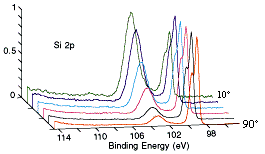For all the experimental details and a more detailed spectral interpretation see the source document : Physical Electronics, Inc. (PHI) Application Note: Angle-Dependent Analysis of a Silicon Wafer with a Native Oxide

Note: in this instance the emission angle is measured with respect to the surface plane (i.e. 90ş corresponds to photoelectrons departing with a trajectory normal to the surface, while 10ş corresponds to emission at a very grazing angle.